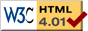Nav: [home] > [sim] > [ljfluid]

## Simple molecular dynamics simulation of a Lennard-Jones fluid

This is about a simple molecular dynamics simulation of a fluid using the Lennard-Jones potential. Phase transitions can be observed by comparing the pair correlation functions at differnet temperatures.

### News

08/2005: Updated Version 0.9
This version has support for plotting the current temperature, energy and pressure as it evolves over time. This also helps finding the time the equilibrium is reached. Furthermore, more images were added here.
08/2004: Initial Release
Initial release of the molecular dynamics sim to compute the pair correlation function.

### What is being done

The simulation is very easily explained: There are N particles in a 3dim box of size L (hence the particle density rho=N/L3). Initially the particles are put randomly inside the box and are given random initial velocities. (Actually, the particles are not placed completely random to make sure that they do not happen to lie too tight together. And it is made sure that the initial velocities sum up to zero so that the total momentum is 0.)

The time evolution of the system is simulated by computing the positions of all the particles in the box. A speed-optimized Verlet algorithm is used for numerical ODE integration. (The algorithm does not need to be very accurate in the particle positions but should conserve total momentum and speed.) The box has periodic boundary conditions which means that particles which leave the box on one side re-enter on the opposite side.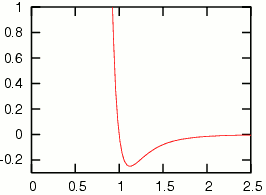The particles interact by the Lennard-Jones potential which looks like V(r)=V0(r-12-r-6) where r is dimensionless. (In particular V(inf)=0, V(0)=+inf and there is a minimum at V(r=21/6)=-V0/4) Hence, the Lennard-Jones potential is very repulsive when the particles approach each other at a distance less than r=0.9 but attractive in the range r=1..+inf with an attraction max at r=21/6. In the simulation, the potential is set to 0 for distances bigger than a certain cutoff raduis (normally 2.5 to 3) to limit the "force range". Note that the periodic boundary conditions make force calculations a bit tricky since particles can interact across the walls of the box. The graph on the left shows V(r)/V0.

The temperature of the fluid (or gas) inside the box is indirectly set via the initial particle velocities: The larger the velocities the higher the temperature.

The main purpose of the simulation is to compute the pair correlation function (PCF) of the particles which is averaged over a large number of time development steps. (Of course, the periodic boundary conditions are also taken into account when computing the PCF.) Furthermore one can visualize the pressure, temperature and energy over time.

### The simulation

For the actual simulation, I wrote a small program which does not require any additional libraries, just a C++ compiler and GNUPlot(1) for the graphics. It compiles natively under Linux but porting to other operating system should not be hard.

The full source code is provided here so that everybody can do his own experiments. For more information on the simulation and where to tune the parameters refer to the comments in the source code.

The whole simulation is done in "natural" scale units, i.e. the potential V(r) is as specified above with V0=4, all particle masses are set to 1, etc. (If you are interested in the details of natural units, look into fluid.cc, around line 50.)

 Source: ljfluid.tar.gz   [10kb gzipped source tarball] Version: 0.9b   (2006-03-26) Author: Wolfgang Wieser   (report bugs here) License: GNU GPL (Version 2) Requires: C++ (gcc recommended), gnuplot

In order to run the simulation, compile and start the fluid program and pipe the output into gnuplot. Alternatively one can use make run which does pretty much the same. Gnuplot will plot the data into particle.ps which can be viewed e.g. using gv. (Gv can be used while the sim is running when at least one page was dumped so far.)

While the simulation is running, it outputs status information to the terminal (stderr) which looks as follows:

```  nskip=0+414884, ncalc=84616, border=97.1%; rmin=0.861659; max_s=0.01298
Iter: |P|=2.1e-13, E_kin=3979.8, T=2.6532, E_pot=-4352, E/N=-0.372208, p=15.9948
```

This tells you the following: In the last iteration, pair interaction between ncalc=84616 distinct particle pairs was calculated while nskip=414884 pairs were skipped (due to cutoff). These values always sum up to N/2*(N-1). 97.1% of all particles required special treatment because they were near one of the box borders. The minimum distande between two particles was rmin=0.86 and the maximum sigma (standard deviation) in the PCF accumulated so far is max_s=0.01298. The simulation will stop when this value drops below pcf_sigma.

Furthermore, the simulation just finished the 2200th iteration. The total momentum of all particles is |P|=2.1e-13. This was initially normalized to zero and should stay very small over all the sim. If it does not, you are in trouble. The total kinetic and potential energies are E_kin=3979.8 and E_pot=-4352, respectively, so the total energy per particle is E/N=-0.37. The current temperature is T=2.65 and the current pressure is p=15.99.

### Contents of particle.ps

The output file particle.ps contains the following: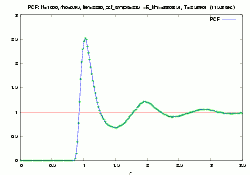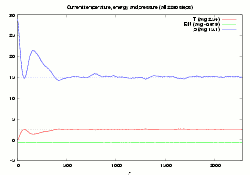First, there are several pages of simulation dumps which are mainly interesting for debugging and seeing what is going on and represent the simulation in action and no final results: Red crosses display an x-y dump (z axis projected) of all particles. The curve on the top is the current PCF. The blue curve is the averaged PCF with error bars. This is not displayed for the first pcf_skip cycles. The curve on the bottom is normalized particle velocity versus particle index and can be used to detect errors in the simulation like excessively fast particles due to too large time steps. Then, there is one page with the computed PCF which is the most important final result of the simulation. The title displays the number of particles (N), the density (rho), the number of iterations performed (iter), the number of PCF samples taken (pcf_samps), the averaged kinetic energy (), the (averaged) temperature (T) and finally how many seconds the complete simulation took. Note that the PCF should always start at zero and converge to 1 for large radi. Finally, there are 3 pages which display the pressure, the total energy per particle and the temperature as a function of simulation time. These 3 pages display essentially the same functions but the first page only displays the first 1% of the sim, the second one the first 10% and the last one displays the complete sim. The dotted lines are the averages of the three curves (real values on the right top); note that collecting values for the average is started after pcf_skip iterations. The most important thing is to ensure that pcf_skip is so large that it skips the initial iterations where the equilibrium state is not yet reached. Also, the total energy per particle (green curve) should stay constant throughout the sim (energy conservation) unless COOL_FACT is switched on, of course.

### Example results

These are some simulations I did as examples for all 3 states of the fluid: solid, liquid, gaseous. I always used N=1000 particles and a density rho around 1; detailed parameters are specified below.
Click on the images to enlarge.

 Solid Liquid Gaseous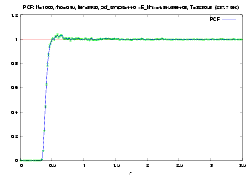First, the fluid in solid state: One can see the high peak which is near the minimum of the Lennard-Jones potential (r=21/6=1.1225). There are no particles less distant than 1 since the potential is repulsive in this area. One can see a double peak near r=2 which are the second- and third-next particles and a further peak near 3 for the fourth-next neighbours. The liquid state with an inital speed around 1 (0.8; any small value will do, even 0 as long as active cooling using COOL_FACT is switched off). Note the characteristic smooth "damped wave" (sort of). It represents the transition between the highly ordered solid state and the unordered gas state. Finally, the gas state. Particles can approach each other more closely (like r=0.4) since their kinetic energy is very large. Note that there is very little distance-related correlation: The PCF changes pretty sharply from 0 to 1 which is a sign for the lack of order in that phase.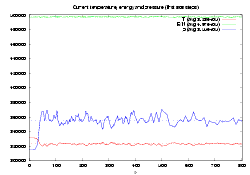Due to cooling, the energy is not conserved and drops to negative (binding) energy. Temperature drops to nearly zero, and pressure settles down to a minimum given by the Lennard-Jones potential and the density. (One can have negative pressure when the particle density rho is so small that the particle distance is smaller than the radius of the minimum in the LJ potential.) In the T,E,p versus time plot, one can see that equilibrium is reached after about 400 simulation cycles. Then, all parameters stay fairly constant. Due to the much larger speed of the particles (speed 1000 times larger than for liquid but time steps only 50 times smaller time step), the equilibrium is reached much faster (about 50 cycles). However, thermal fluctuations are much larger. Parameters: ``` int pcf_samples=8; int pcf_skip=10000; int dumpfreq=100; int currfreq1=10; int currfreq0=2; double pcf_sigma=0.01; sim.Initialize(/*N=*/1000, /*dt=*/1e-3, /*rho=*/1.05, /*cutoff_r=*/3.5, /*initial_v=*/10); sim.InitializePCF(200,3.5); ``` The initial velocity was set to 10 and the system was cooled down during the first 10000 iterations (dt=1e-3) using COOL_FACT *0.997. Parameters: ``` int pcf_samples=8; int pcf_skip=400; int dumpfreq=100; int currfreq1=10; int currfreq0=2; double pcf_sigma=0.01/1.2; sim.Initialize(/*N=*/1000, /*dt=*/0.5e-3, /*rho=*/0.95, /*cutoff_r=*/3.5, /*initial_v=*/0.8); sim.InitializePCF(300,3.5); ``` Parameters: ``` int pcf_samples=8; int pcf_skip=400; int dumpfreq=100; int currfreq1=10; int currfreq0=2; double pcf_sigma=0.01; sim.Initialize(/*N=*/1000, /*dt=*/1e-5, /*rho=*/0.95, /*cutoff_r=*/3.5, /*initial_v=*/1000); sim.InitializePCF(200,3.5); ```